## AppKiwi - Download Mobile Apps on PC & Mac# Microsoft Math Solver on PC/Mac

Author: Microsoft Corporation

Score: 4.7

OS: Windows, OS X, 5.0 +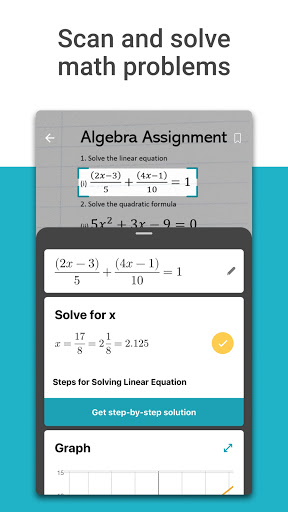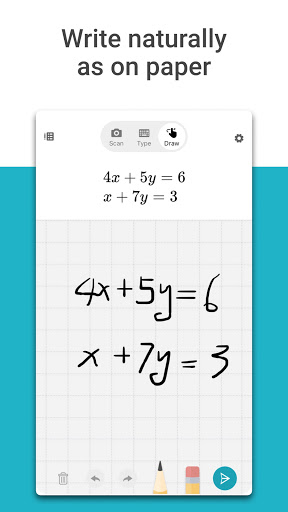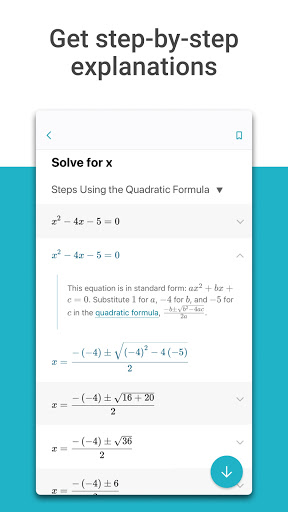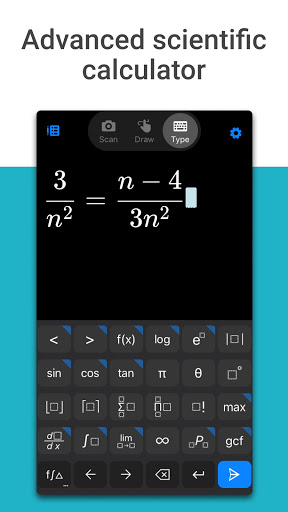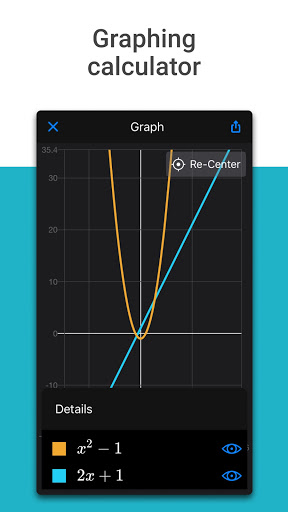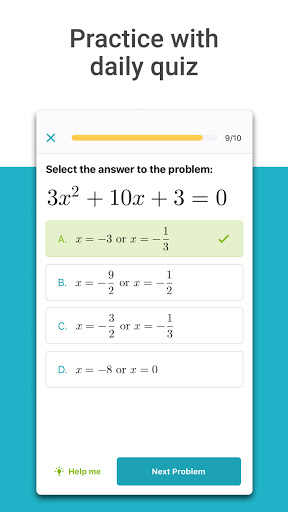##### About Microsoft Math Solver
Microsoft Math solver app provides help with a variety of problems including arithmetic, algebra, trigonometry, calculus, statistics, and other topics using an advanced AI powered math solver. Simply write a problem on screen or use the camera to snap a math photo. Microsoft Math problem solver instantly recognizes the problem and helps you to solve it with ⚡FREE Step-By-Step Explanations⚡, interactive graphs, similar problems from the web and online video lectures. Quickly look up related concepts. Get help with your homework problems and gain confidence in mastering 💯 the techniques ⚡It is absolutely FREE and No Ads!⚡

## HIGHLIGHTS

● ✏️ Write an equation on screen as you naturally do on paper
● 📷 Scan printed or handwritten math problem
● ⌨️ Type and edit using advanced scientific calculator
● Get interactive Step-by-Step explanations 💡 & Graphing calculator 📈
● Import images with equations from gallery 🖼️
● Scan and Solve Worksheets with multiple problems
● 🔎Search the web for similar problems and video lectures
● Try word problems
● Scan and plot x-y data tables for linear/non-linear functions
● Learn mathematics in your language - supports Chinese, French, German, Hindi, Italian, Japanese, Portuguese, Russian, Spanish, and many more

## SUPPORTED PROBLEMS

● Elementary: arithmetic, real, complex numbers, LCM, GCD, factors, roman numerals
● Pre-Algebra: radicals and exponents, fractions, matrices, determinants
● Algebra: quadratic & simultaneous equations, inequalities, rational expressions, linear, polynomial and exponential graphs
● Word problems on mathematical concepts, number theory, probability, volume, surface area
● Basic Calculus: Summations, Limits, derivatives, integrals
● Statistics: Mean, Median, Mode, Standard Deviation, permutations, combinations

Find out more about Microsoft Math Solver app on our website 👉 Link: https://math.microsoft.com

📧 Contact us at email: mathsupport@microsoft.com We look forward to your feedback.
##### Recent Updates - Version 1.0.186
• - Bug fixes and performance improvements